# 数据挖掘入门系列教程（三点五）之决策树

2019/04/10 10:10

## 数据挖掘入门系列教程（三点五）之决策树

### 决策树（Decision Tree）介绍

Decision Tree是一类较为常见的机器学习的方法。它既可以作为分类算法，也可以作为回归算法。

• Q：性别要求？
• A：不是女的不要。
• Q：年龄要求？
• A：大于我3岁的不要
• Q：专业要求？
• A：非CS不要
• ……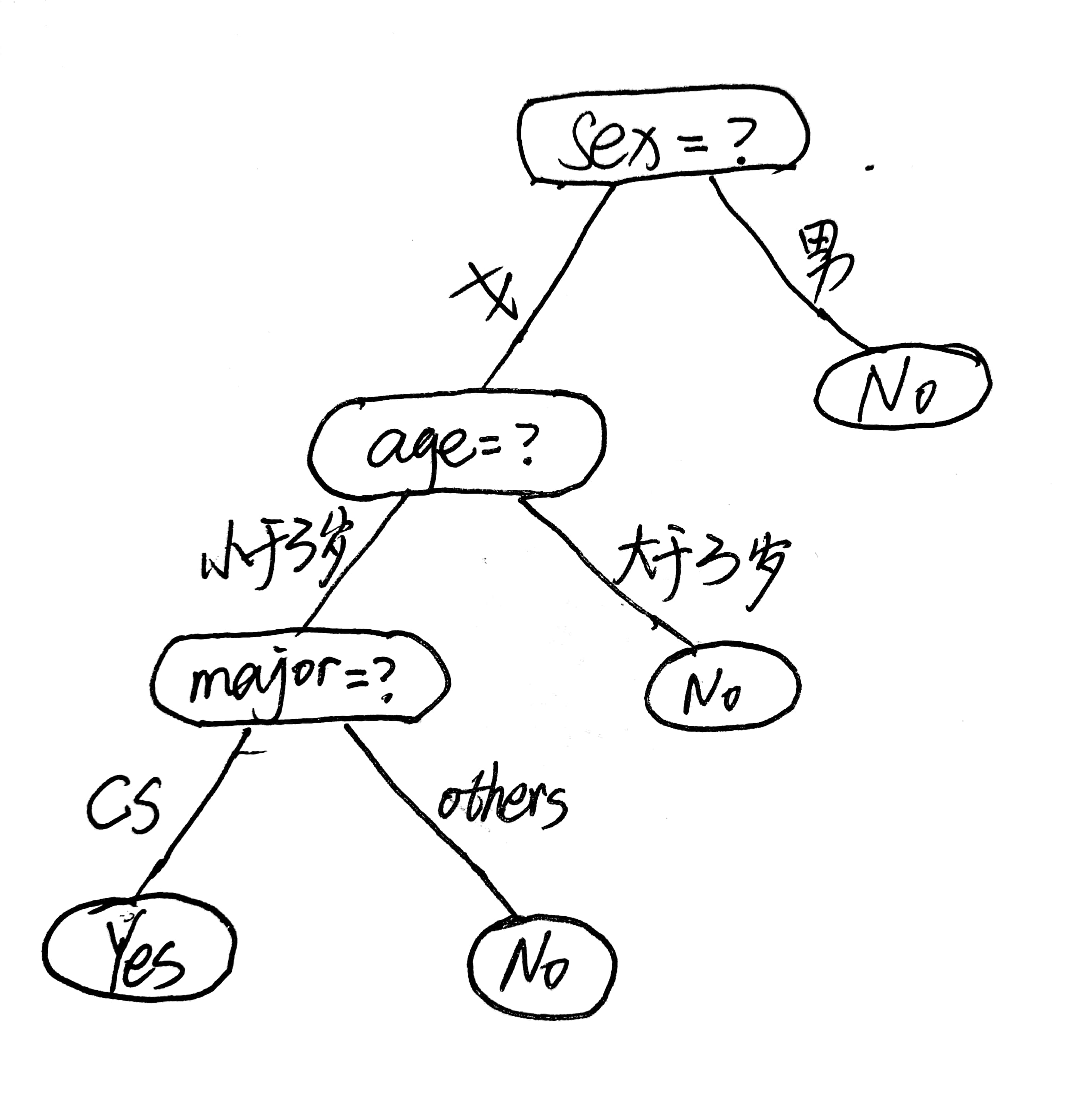• 根节点：包含样本的全集
• 内部节点：对应特征属性测试
• 叶节点：代表决策的结果

### 结点的选择

“信息熵”（information entropy）：可以度量样本集合中的“纯度”。 在信息世界，熵越高，表示蕴含越多的信息，熵越低，则信息越少。 而根节点需要包含所以的样本，则根结点的熵最大

#### 信息熵（Information Entropy）

$Ent(D) = - \sum_{k=1}^{n}p_klog_2p_k\ Ent(D)越大，则D的纯度越小，也就是说集合D越混乱。$

#### 信息增益（information gain）

$Gain(D,a) = Ent(D) - \sum_{v=1}^V\frac{|D^v|}{|D|}Ent(D^v)$

### 决策树生成算法流程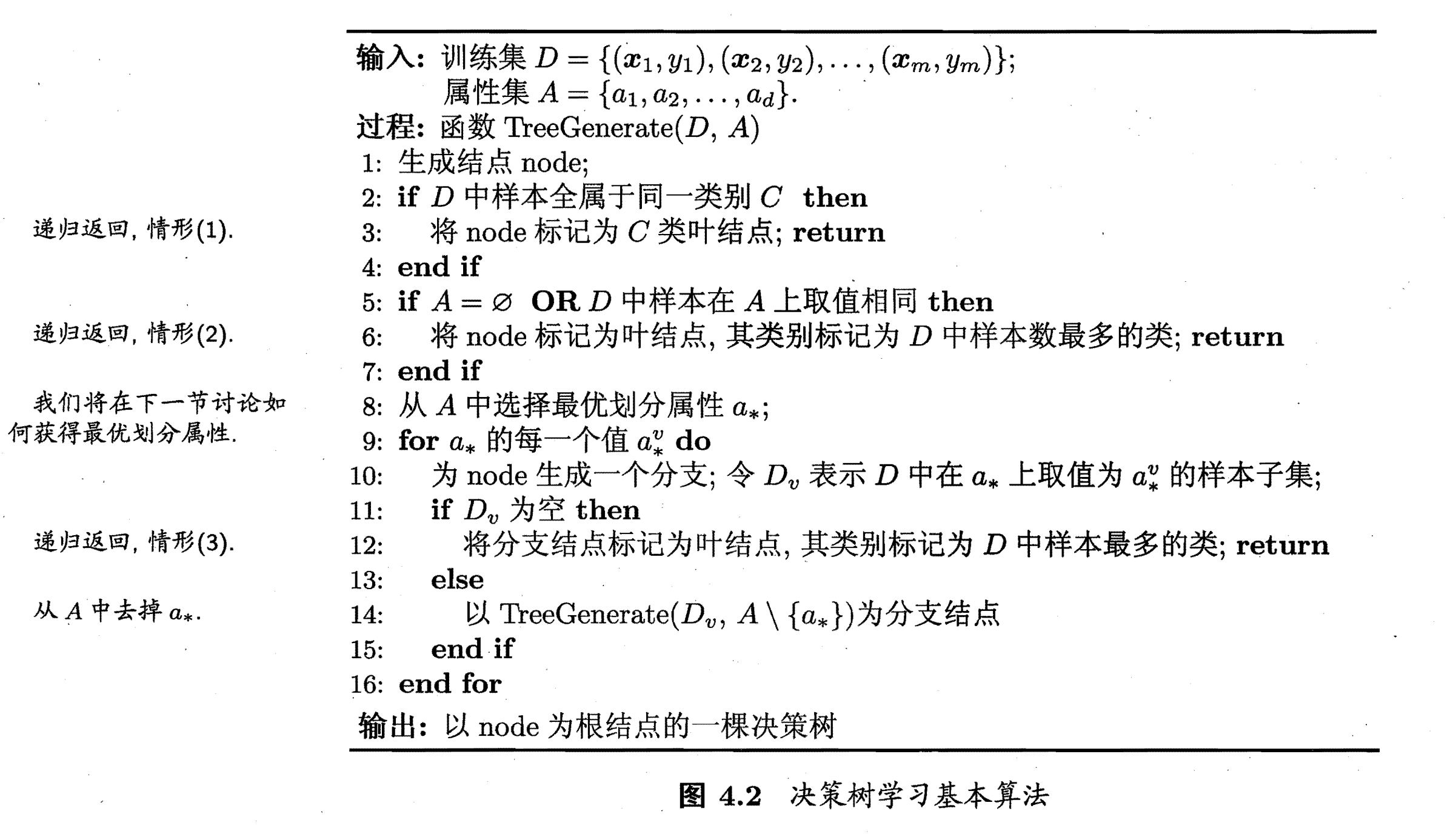• 当前结点包含的样本全属于同一类别，无需划分
• 当前属性集为空，或是所有样本在所有属性上取值相同，无法划分
• 当前结点包含的样本集合为空，不能划分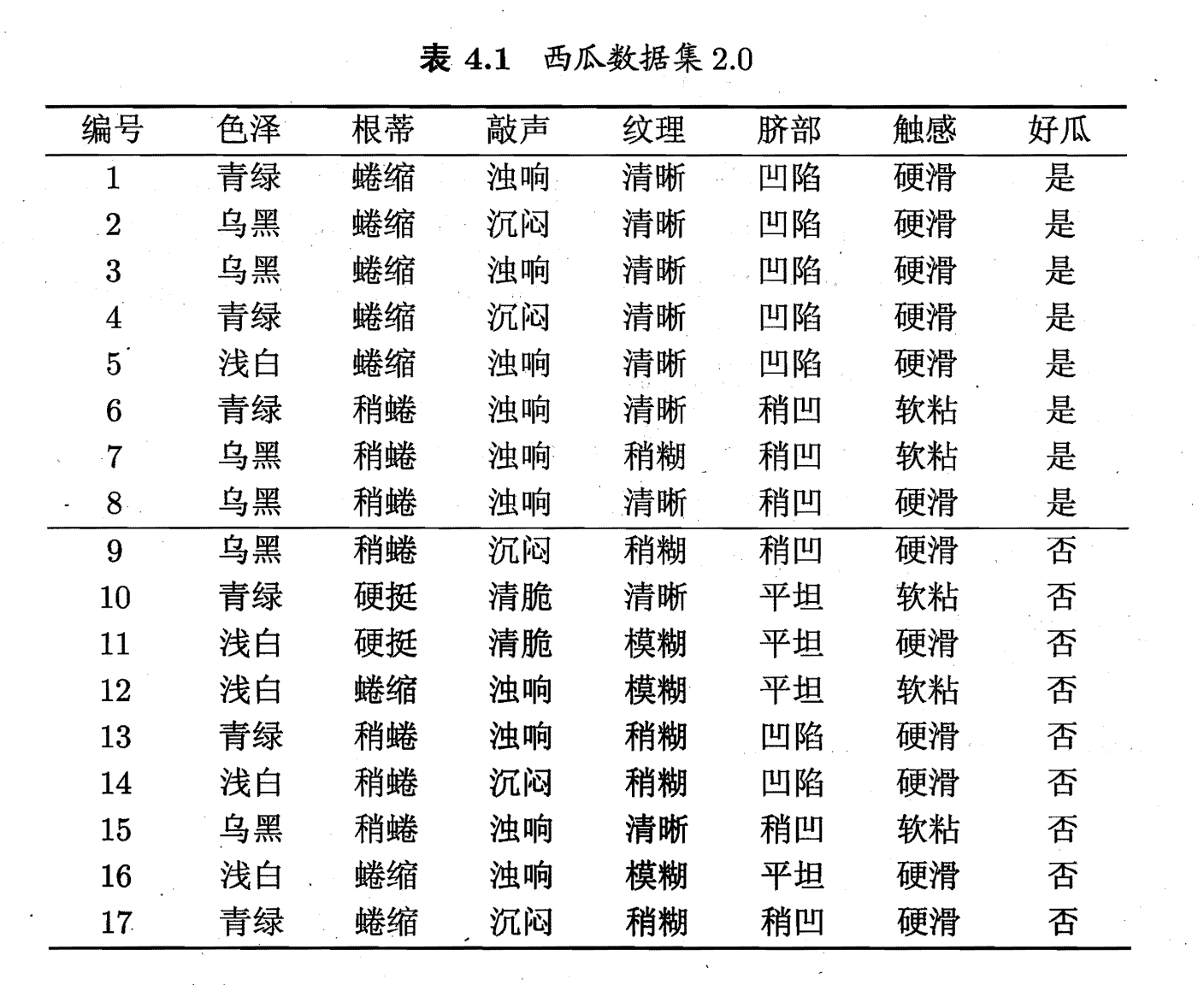$Ent(D^1)=-(\frac{3}{6}log_2\frac{3}{6}+\frac{3}{6}log_2\frac{3}{6}) = 1.00 \ Ent(D^2)=-(\frac{4}{6}log_2\frac{4}{6}+\frac{2}{6}log_2\frac{2}{6}) = 0.918 \ Ent(D^3)=-(\frac{1}{5}log_2\frac{1}{5}+\frac{4}{5}log_2\frac{4}{5}) = 0.722 \$

\begin{equation} \begin{aligned} Gain(D，色泽) &= Ent(D) - \sum_{v=1}^3\frac{|D^v|}{|D|}Ent(D^v)\ &= 0.99 - （\frac{6}{17}\times 1.00 + \frac{6}{17}\times0.918 +\frac{5}{17}\times0.722） \ &= 0.109 \end{aligned} \end{equation} 同理可以得到： $\begin{equation} \begin{split} & Gain(D，根蒂) = 0.143；Gain(D，敲声) = 0.141；\ & Gain(D，纹理) = 0.381；Gain(D，脐部) = 0.289； \ & Gain(D，触感) = 0.006；Gain(D，色泽)=0.109； \end{split} \end{equation}$ 通过计算可以得到，纹理的信息增益最大，因此他被选为划分的属性如下图：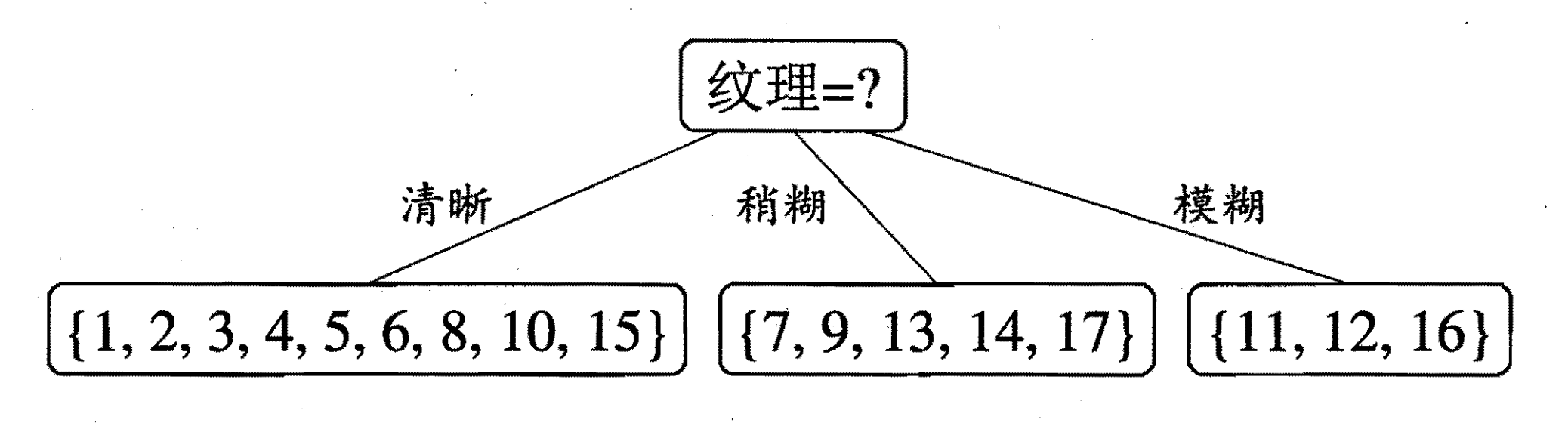$\begin{equation} \begin{split} & Gain(D^1，色泽) = 0.043；Gain(D^1，根蒂) = 0.458；\ &Gain(D^1，敲声) = 0.331； Gain(D^1，触感) = 0.458；\ &Gain(D^1，脐部)=0.458； \end{split} \end{equation}$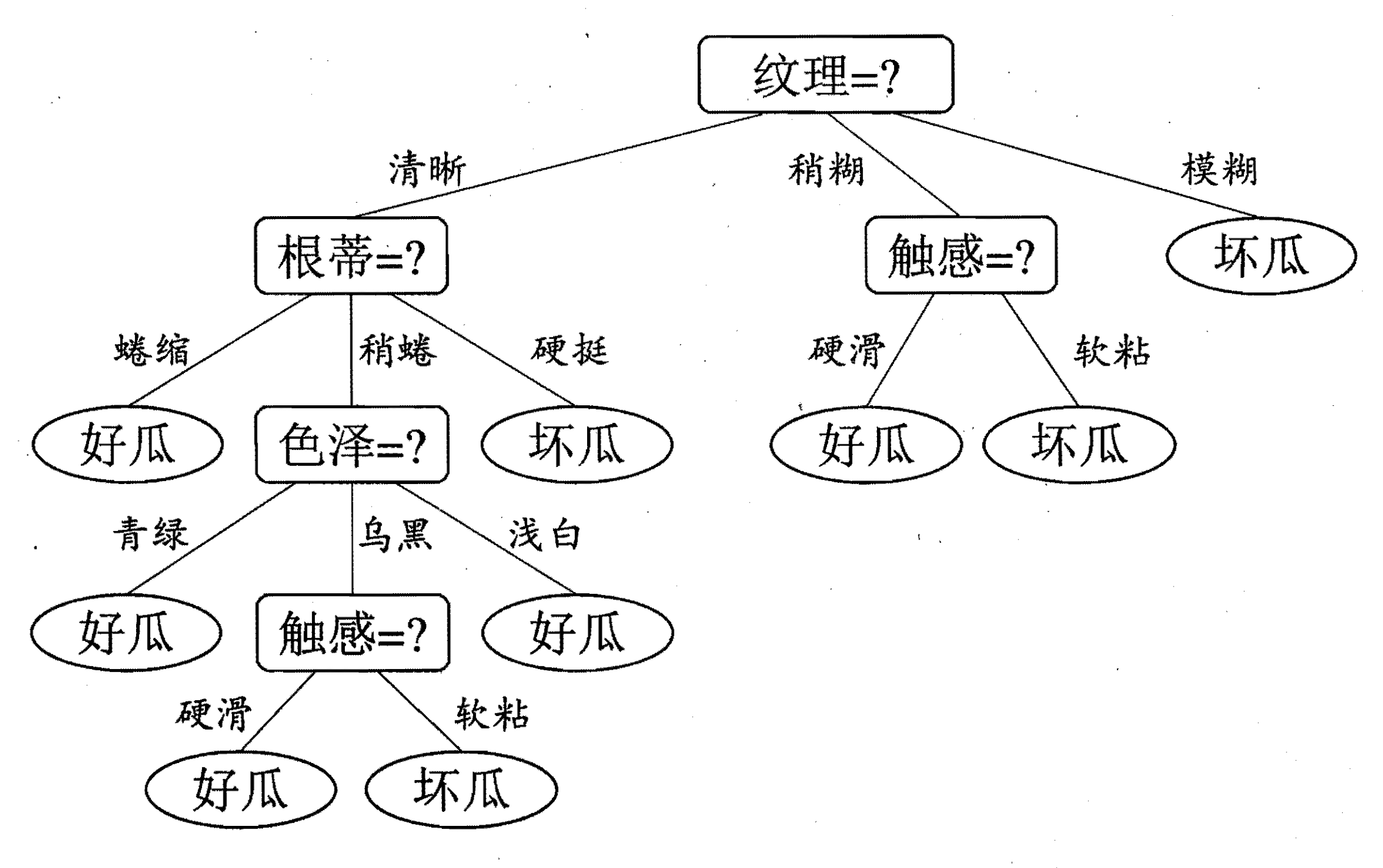### 增益率（Gain Ratio）

$IV(a)$称之为属性$a$的固有值(intrinsic value)，属性$a$的可能取值越多（划分的$D^v$的集合越多），$IV(a)$就会越大。若像编号一样进行划分（每一个划分的集合中只有一个样本），随着编号的增多，$IV(a)$的取值如下图：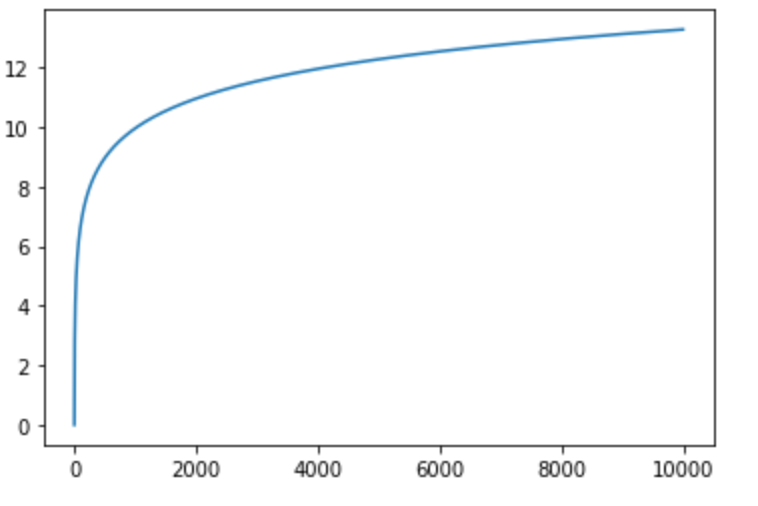### 基尼指数（Gini Index）

$Gini(D)$ 反映了从数据集中随机抽取两个样本，其类别标记不一致的概率。因此， $Gini(D)$越大，则数据集越复杂，纯度越低。

$Gini_index(D,a) = \sum_{v=1}^V\frac{D^v}{D}Gini({D^v})$### 剪枝（pruning）处理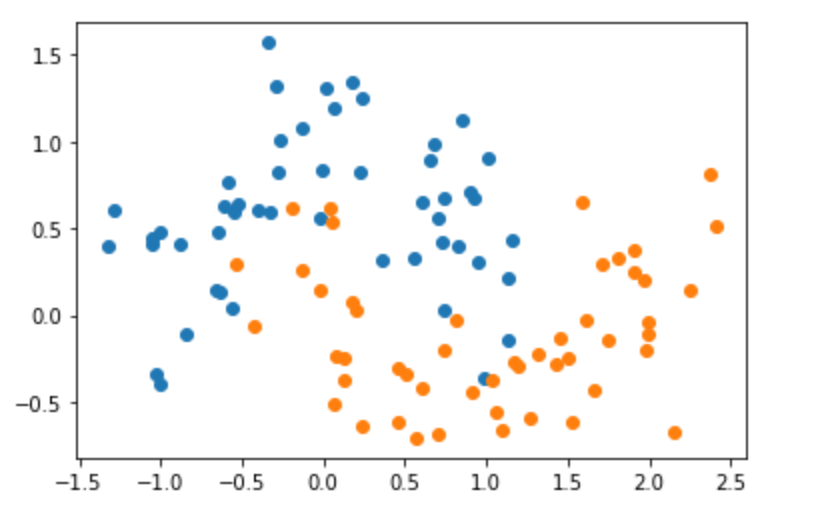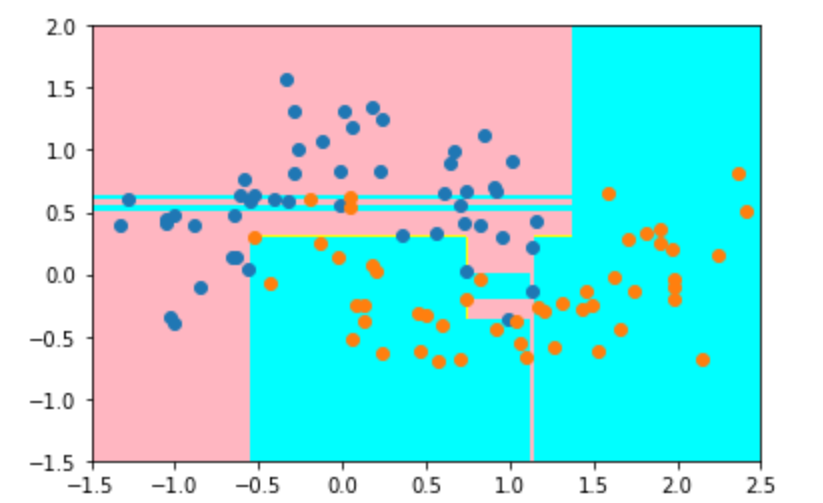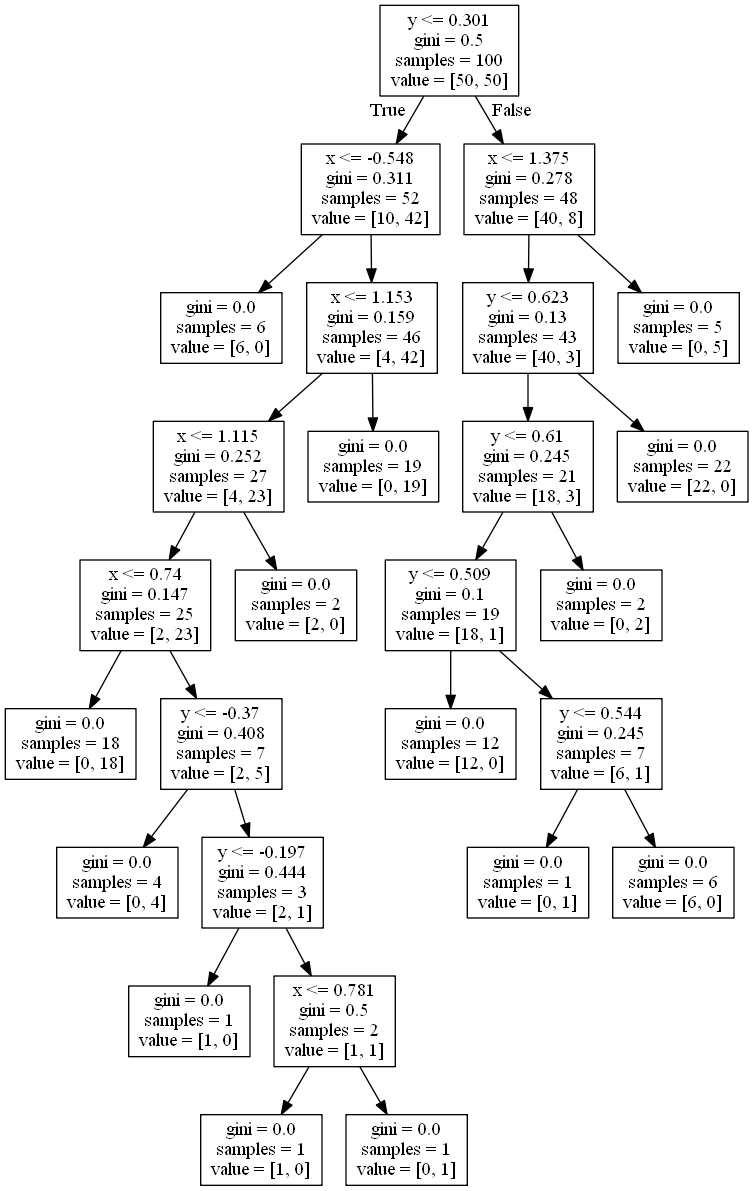• 预剪枝（prepruning）
• 后剪枝（post-pruning）

#### 预剪枝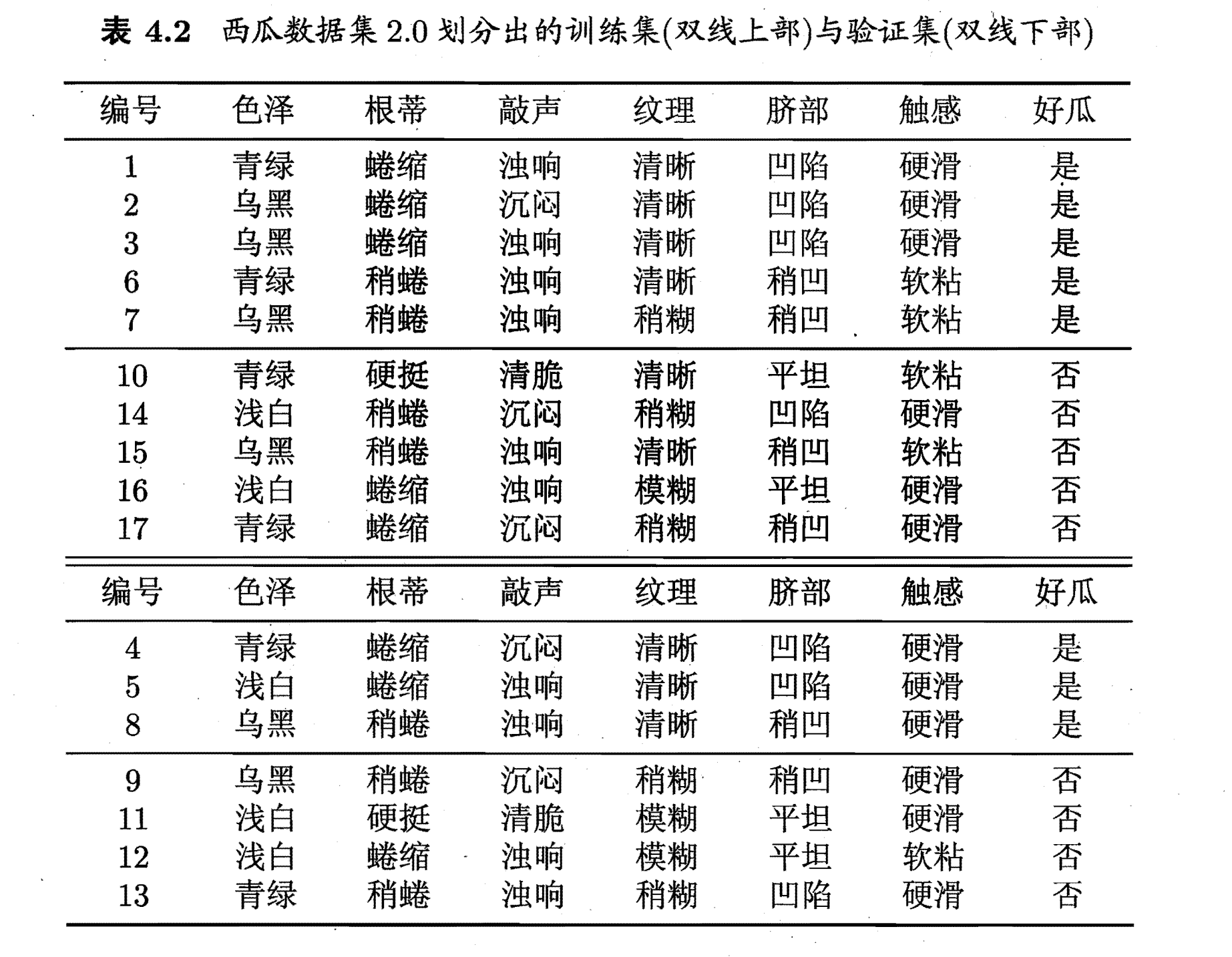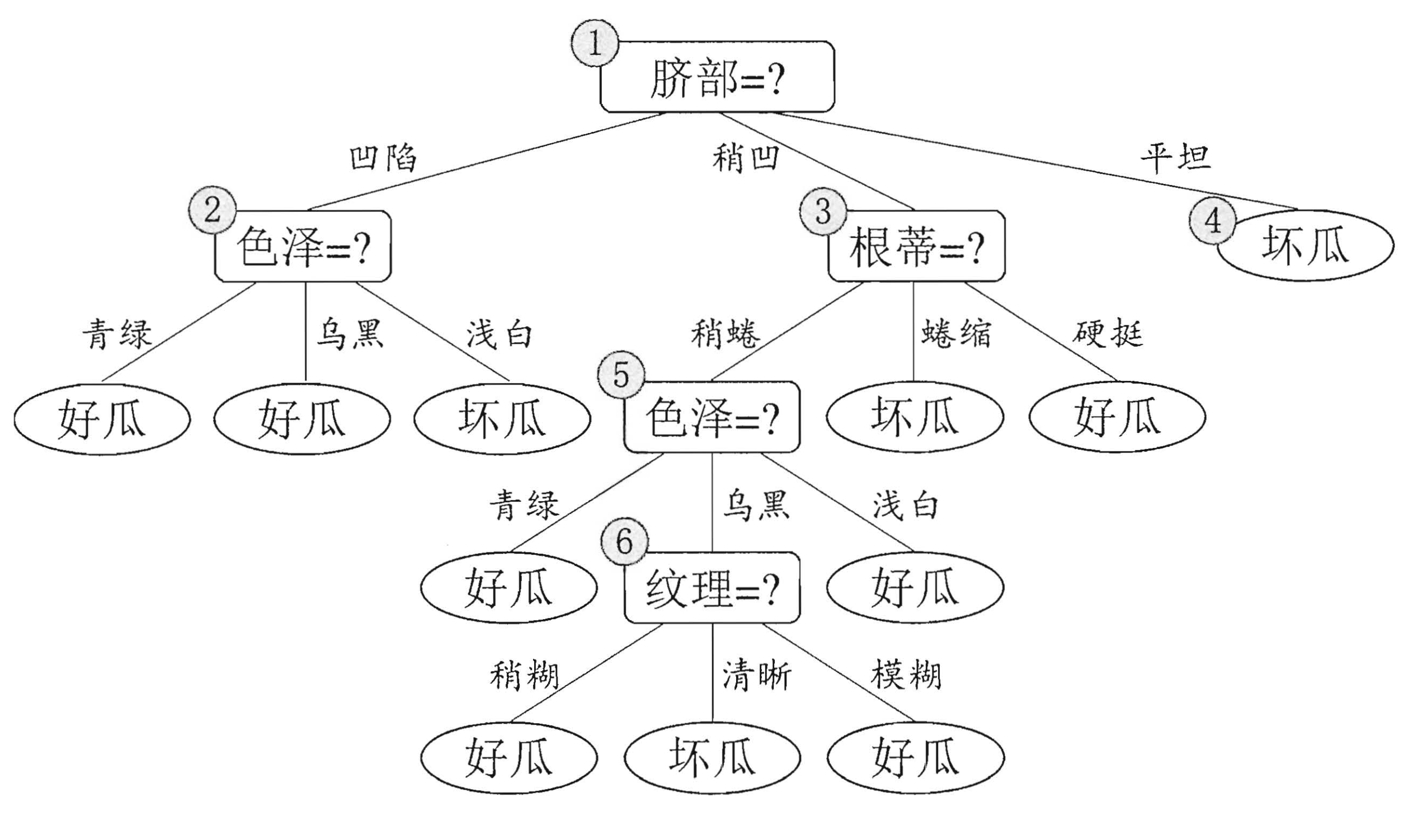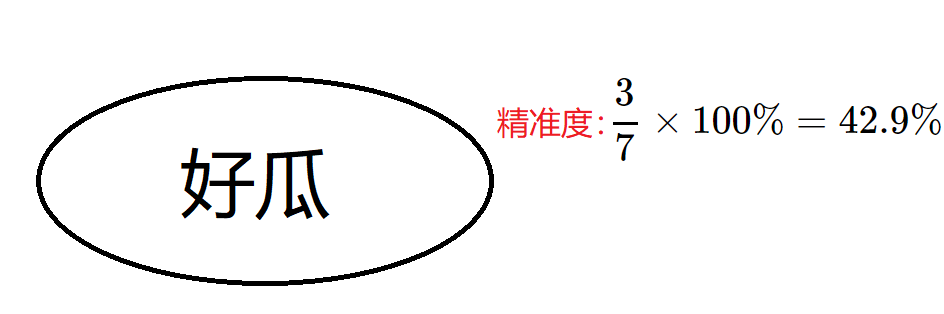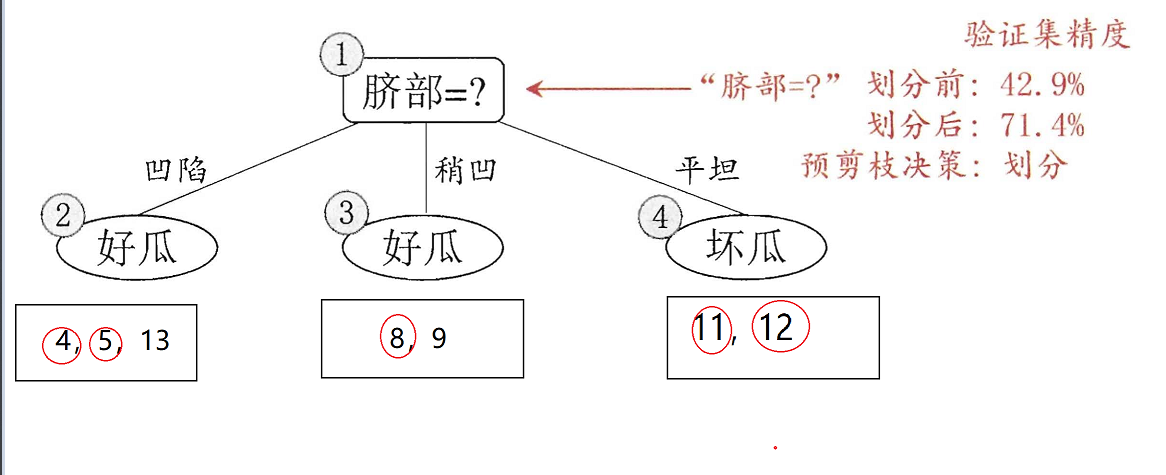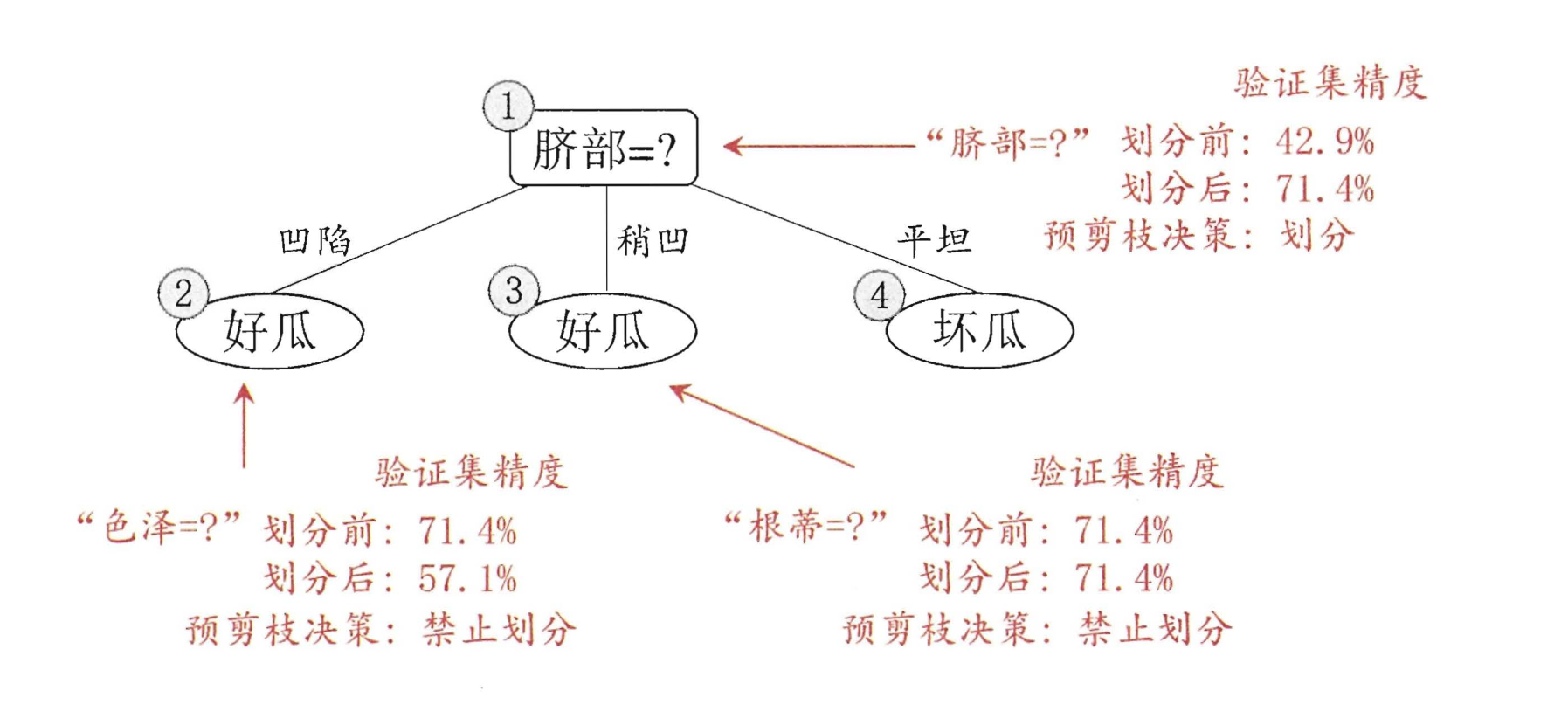#### 后剪枝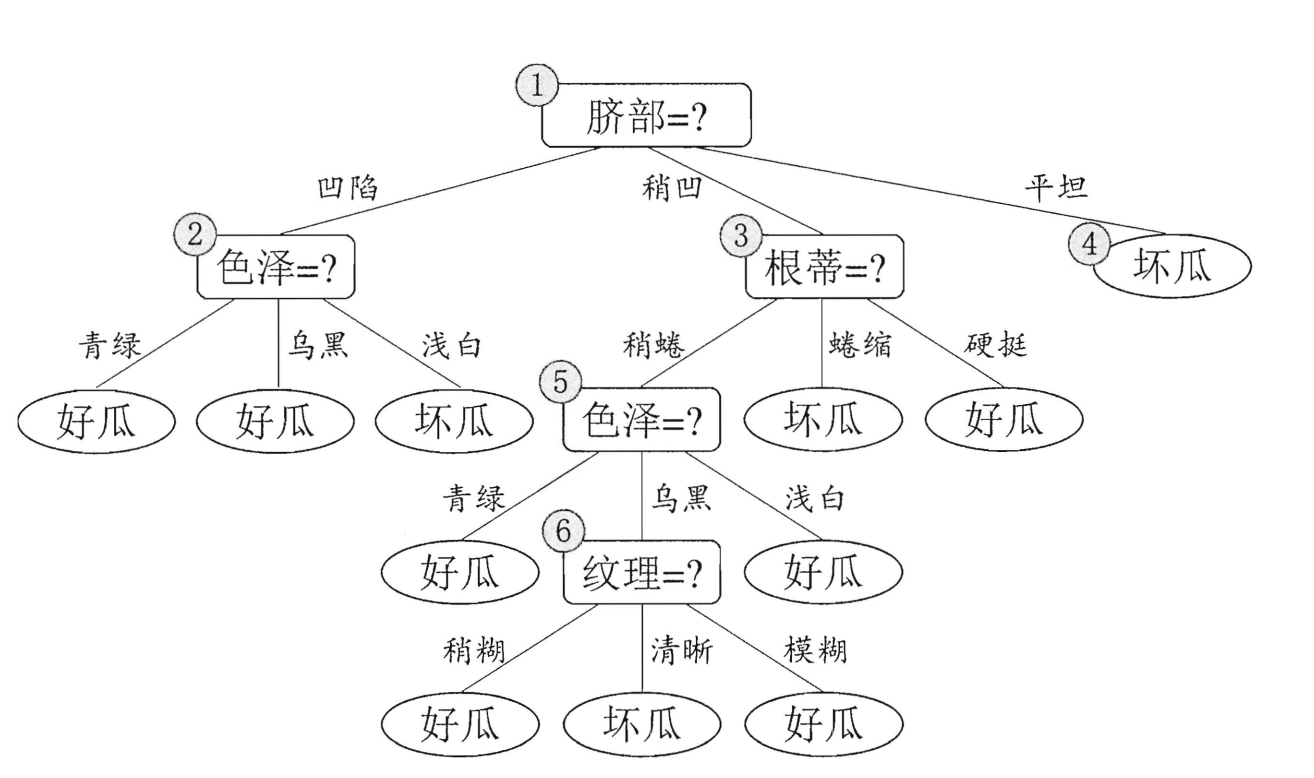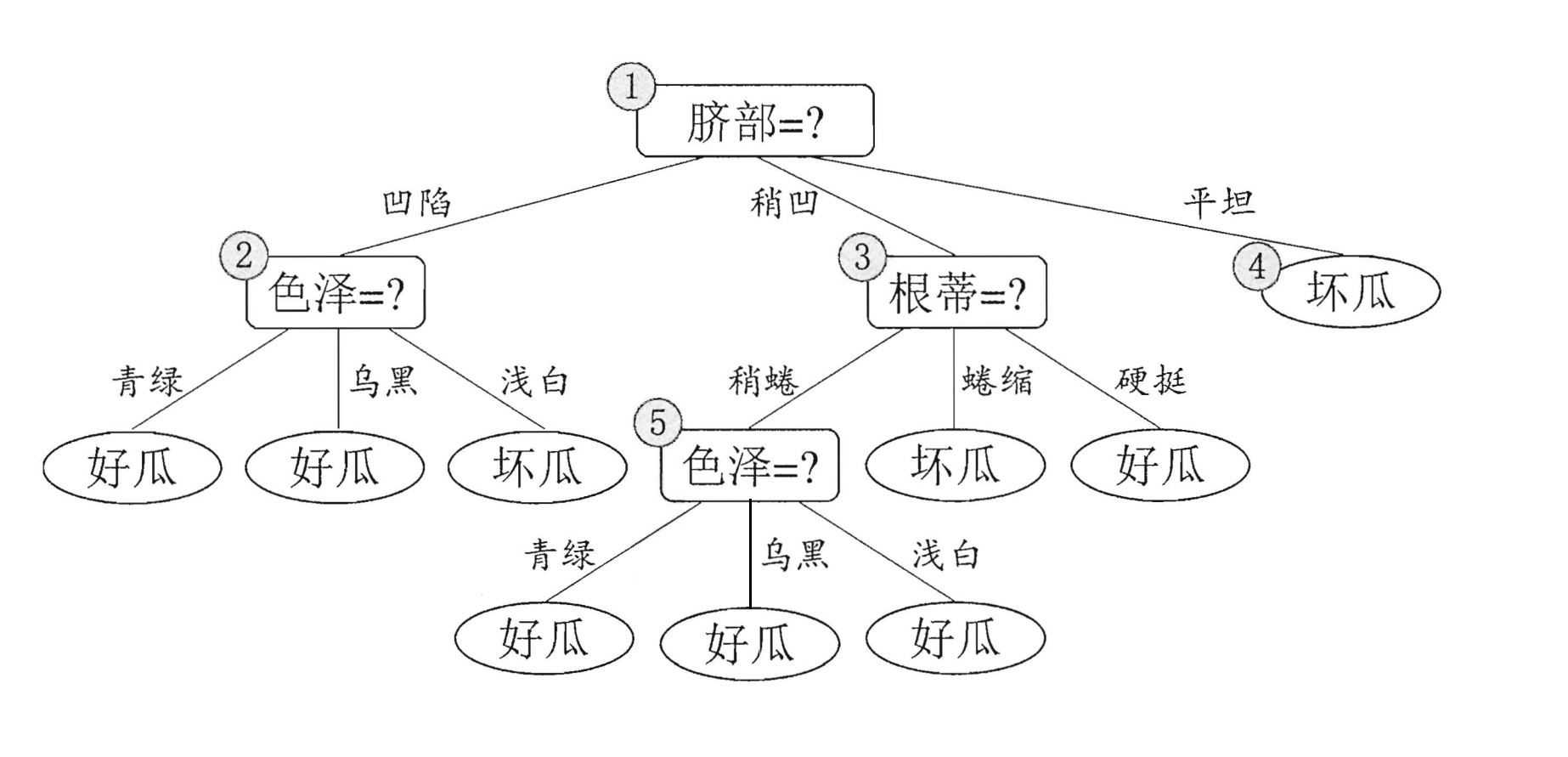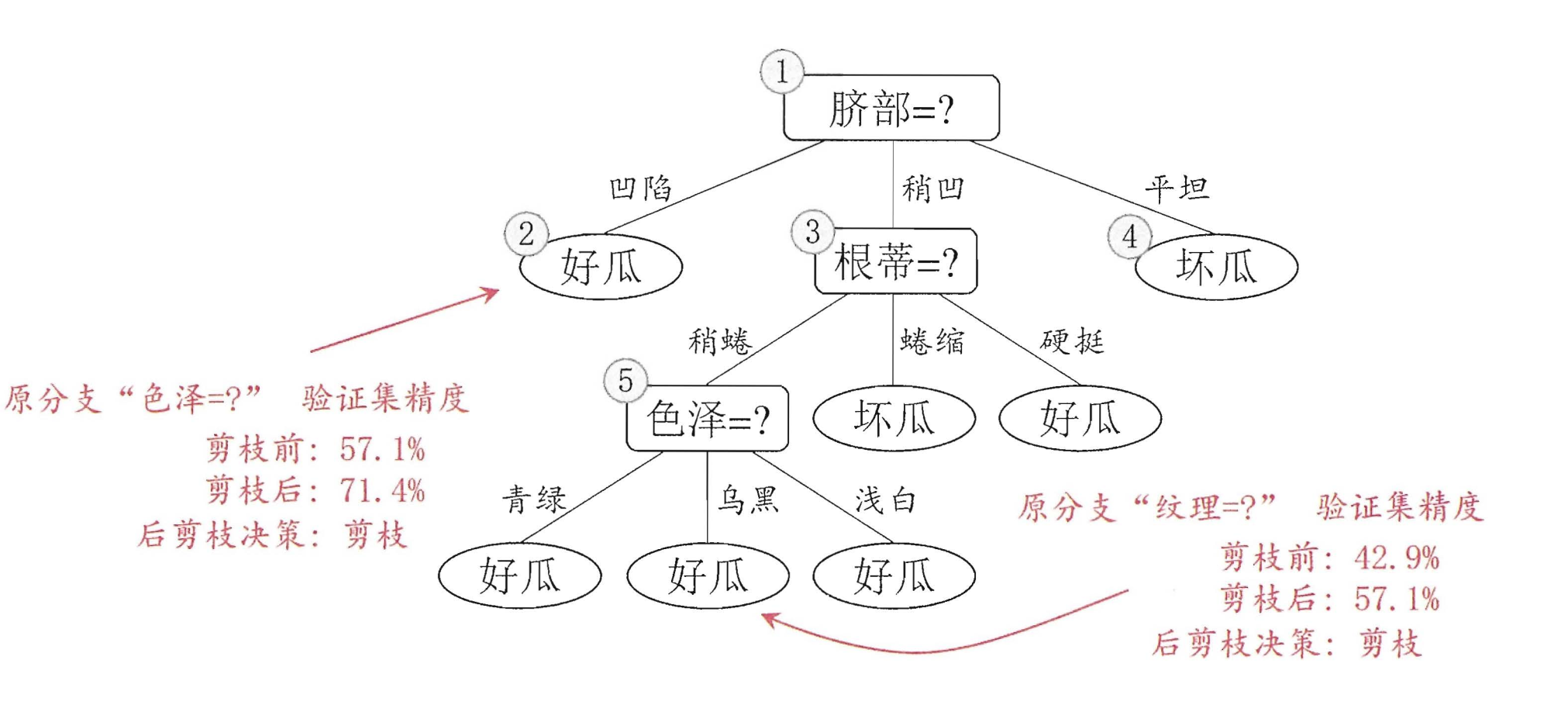### 随机森林（Random Forest | RF）

• 样本随机：Bagging算法
• 属性随机

#### 样本随机

ps：通过以上这种取样得到的集合$D_i$中间可能会有重复的元素（因为是有放回的抽取元素）

#### 属性随机

ps：在这种情况下，$m$个属性中是没有重复的属性的。

#### 随机森林的优缺点

1. 它可以出来很高维度（特征很多）的数据，并且不用降维，无需做特征选择
2. 它可以判断特征的重要程度
3. 可以判断出不同特征之间的相互影响
4. 不容易过拟合
5. 训练速度比较快，容易做成并行方法
6. 实现起来比较简单
7. 对于不平衡的数据集来说，它可以平衡误差。
8. 如果有很大一部分的特征遗失，仍可以维持准确度。

1. 随机森林已经被证明在某些噪音较大的分类或回归问题上会过拟合。
2. 对于有不同取值的属性的数据，取值划分较多的属性会对随机森林产生更大的影响，所以随机森林在这种数据上产出的属性权值是不可信的

ID3 C4.5 CART

### 参考

1. 《西瓜书》决策树——第四章
2. 维基百科：随机森林 Bagging算法### 作者的其它热门文章

0
0 收藏

0 评论
0 收藏
0